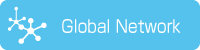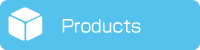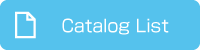1. HOME
2. SUPER UNIT (Analog Command Input, High-Accuracy Type)
3. Continuous and Short-time Rating Range

# SUPER UNIT (Analog Command Input, High-Accuracy Type)Continuous and Short-time Rating Range

Introduce a short-time rating which can operate with a higher output than the continuous rating operation.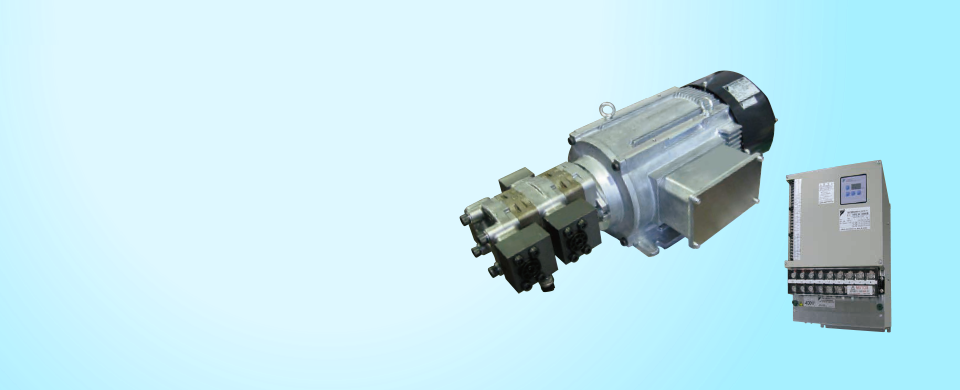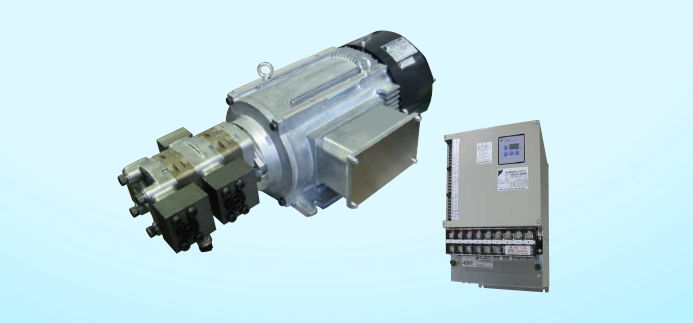## About Continuous and Short-time Rating Range

Analog command input/high-accuracy type SUPER UNITs can run continuously within the continuous rating range given in the pressure - flow rate characteristic charts.

Note, however, that the range of operation can be extended to within the short-time rating range for up to 20 seconds (or 60 seconds for the 400 V single pump type), provided it does not exceed a 20% duty cycle.

Example with Single Pump Type SUT00S20018-20YL-N0340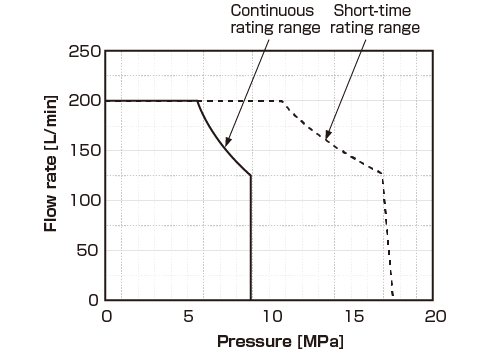Example with Double Pump Type SUT00D13021-10-B-N0321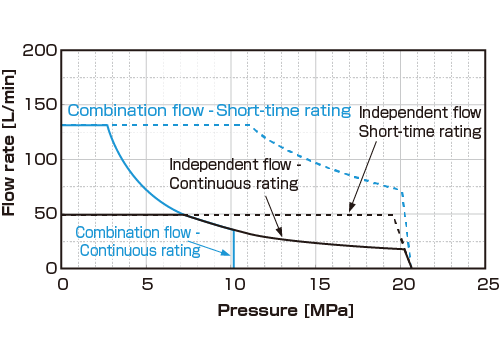## Continuous rating

Continuous operation is possible when the mean hydraulic power obtained based on the pressure and flow rate during operation of 1 cycle is lower than the hydraulic power for the continuous rating range in the figure above and also the root-mean-square of the load pressure is within the maximum pressure for the continuous rating range.

(With the double pump type unit shown in the figure above with independent flow selected, continuous operation with the pressure held at 20.6 MPa is possible. However, for cycles that include pressure holding for 3 minutes or longer, a bleed off circuit equivalent to the capacity of a single pump running at 150 min-1 must be provided at the pump discharge side to cool the pump.)

## Short-time rating

Operation possible for 20 seconds (or 60 seconds with 400 V single pump type)

### ■Reference

#### How to obtain the mean hydraulic power and root-mean-square pressure (example for single pump type)

When load pressure in each process within 1 cycle is Pn(n=1,2,...., n), flow rate is Qn(n=1,2,...., n), and time is tn(n=1,2,...., n)

• Mean hydraulic power＝(P1×Q1/60×t1+P2×Q2/60×t2+…+Pn×Qn/60×tn)/（t1+t2+…+tn)
• Root-mean-square of load pressure＝SQRT((P12×t1+P22×t2+…+Pn2×t2)/(t1＋t2+…+tn)) (Note, however, that if the pump runs above the rated pump rotational speed of 1800 min-1 to provide the control flow rate of Qn, with the load pressure Pn, Pn needs to be converted to the value when the pump is running at 1800 min-1.) When the pump capacity of the SUPER UNIT is qn [cm3], the pump rotational speed Nn is Nn = Qn×103/qn. Therefore, converted pressure Pn = Pn×(Nn/1800)
Note 1:
The procedure for obtaining the mean hydraulic power and root-mean-square pressure with flow-rate-selection specifications (double pump type) is basically the same as above. Please consult us for detailed information.
Note 2:
SQRT above represents square root operation (√). For the continuous rating hydraulic powers for each model, see the pressure - flow rate characteristic charts (P-Q characteristic charts) given in the instruction manual provided separately.